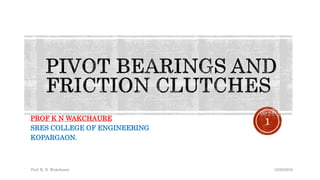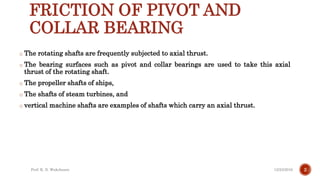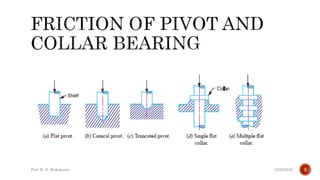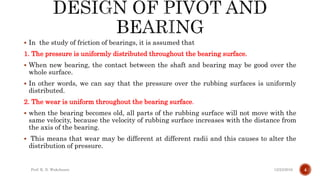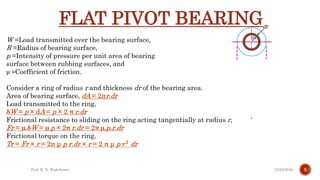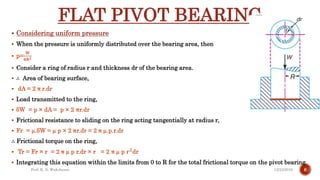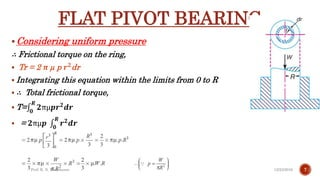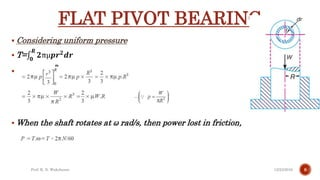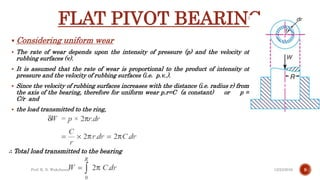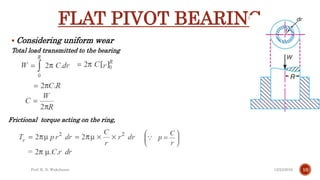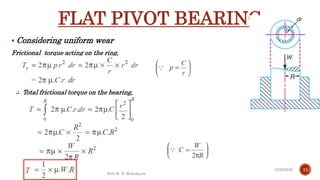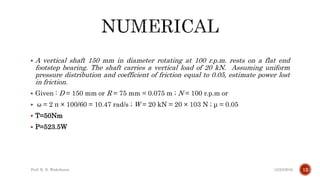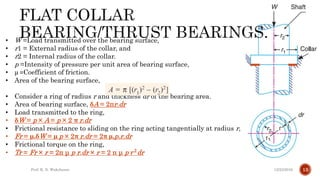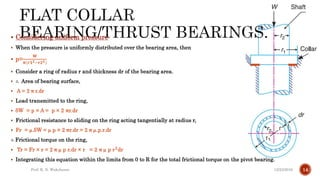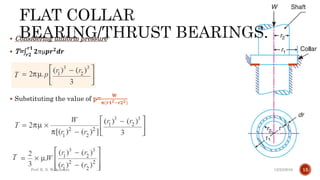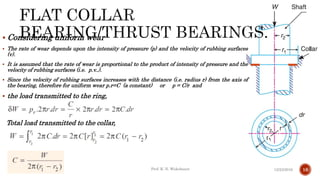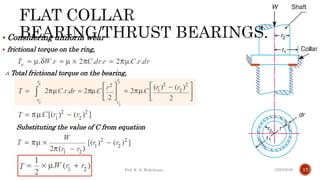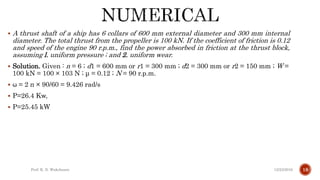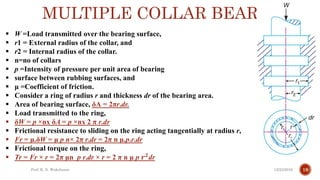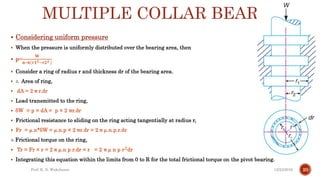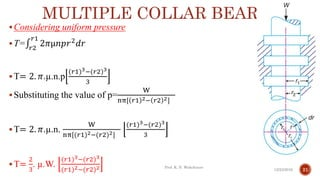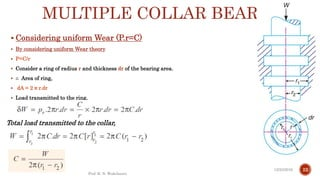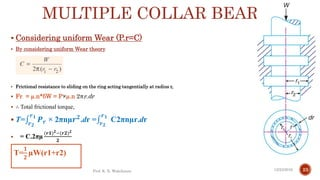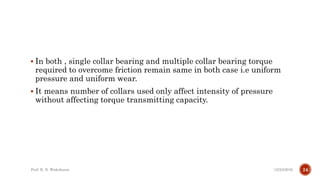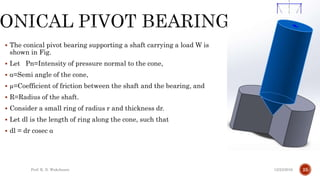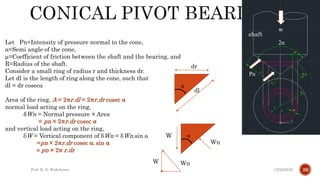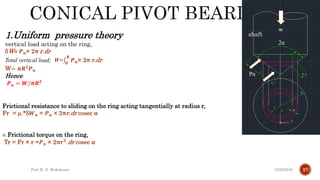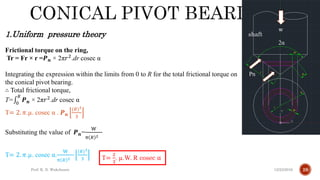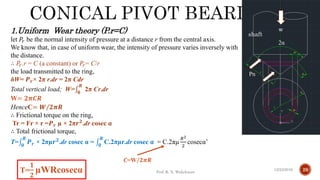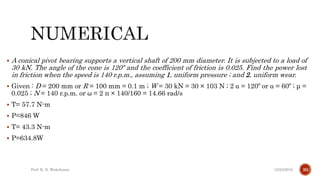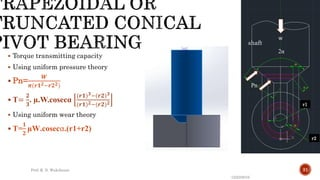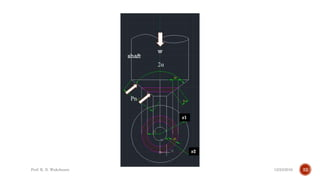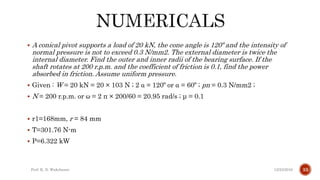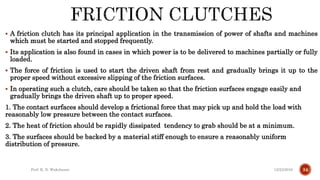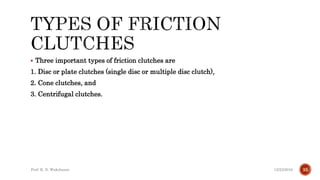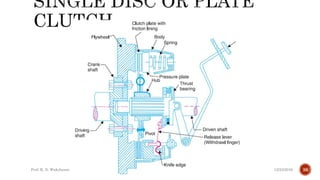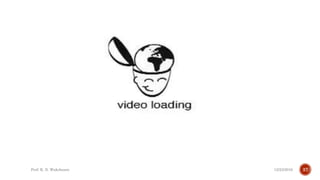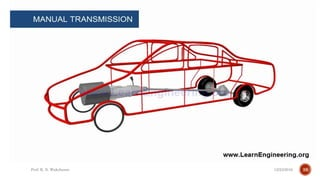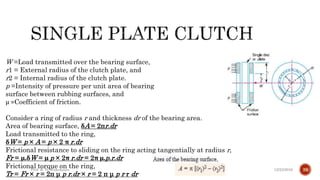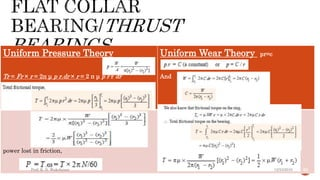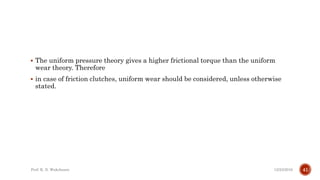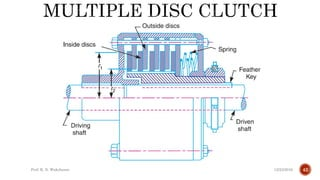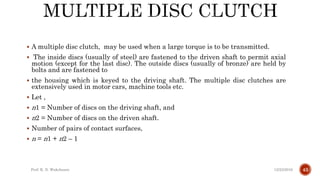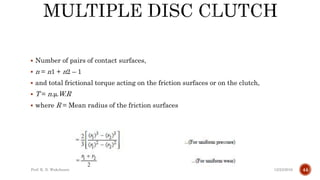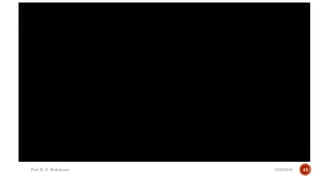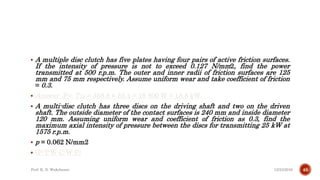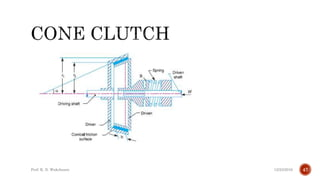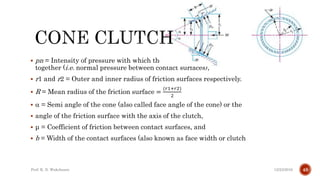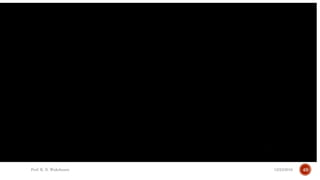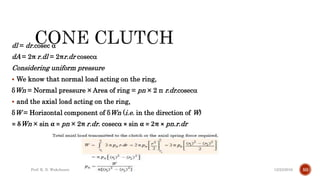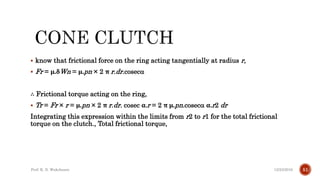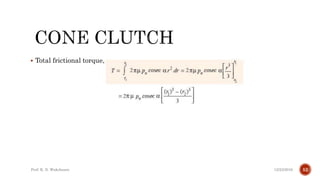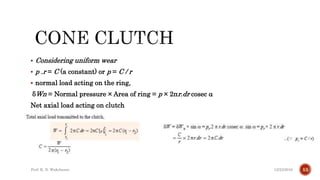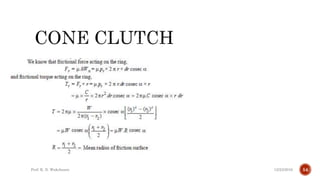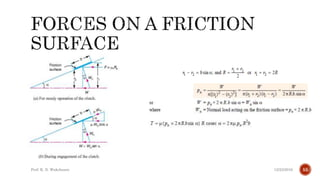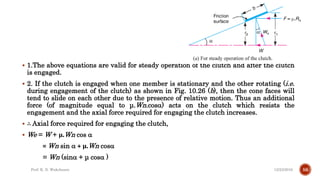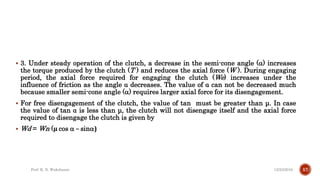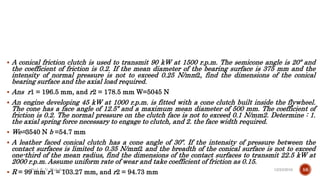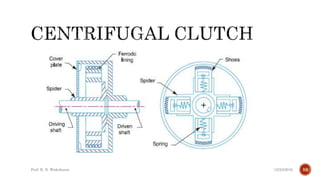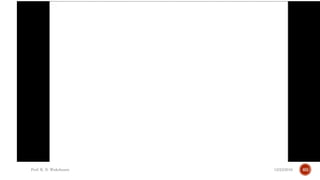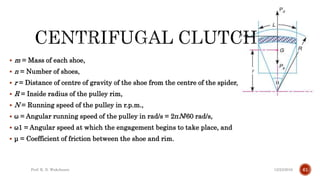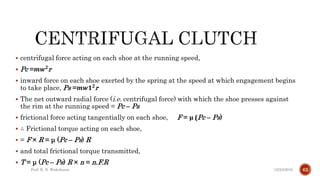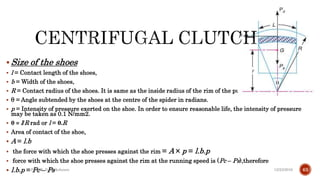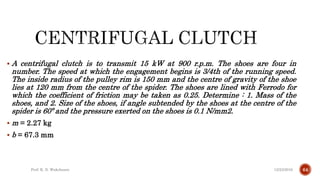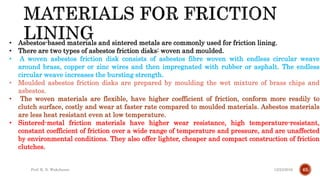1 of 66

## RecommendedPivot bearings and friction clutchesKiran Wakchaure1 s2.0-002980189090003 o-mainhamidkm69IARE_SOM_II_PPT.pdfPrashantKuwarBasic of Clutches, kopling tidak tetapdodi mulyaRelation between load shear force and bending moment of beamssushma chinta

### Pivot and collar friction .pdf

1. PROF K N WAKCHAURE SRES COLLEGE OF ENGINEERING KOPARGAON. 12/23/2016 Prof. K. N. Wakchaure 1
2. FRICTION OF PIVOT AND COLLAR BEARING o The rotating shafts are frequently subjected to axial thrust. o The bearing surfaces such as pivot and collar bearings are used to take this axial thrust of the rotating shaft. o The propeller shafts of ships, o The shafts of steam turbines, and o vertical machine shafts are examples of shafts which carry an axial thrust. 12/23/2016 Prof. K. N. Wakchaure 2
3. 12/23/2016 Prof. K. N. Wakchaure 3
4.  In the study of friction of bearings, it is assumed that 1. The pressure is uniformly distributed throughout the bearing surface.  When new bearing, the contact between the shaft and bearing may be good over the whole surface.  In other words, we can say that the pressure over the rubbing surfaces is uniformly distributed. 2. The wear is uniform throughout the bearing surface.  when the bearing becomes old, all parts of the rubbing surface will not move with the same velocity, because the velocity of rubbing surface increases with the distance from the axis of the bearing.  This means that wear may be different at different radii and this causes to alter the distribution of pressure. 12/23/2016 Prof. K. N. Wakchaure 4
5. FLAT PIVOT BEARING W =Load transmitted over the bearing surface, R =Radius of bearing surface, p =Intensity of pressure per unit area of bearing surface between rubbing surfaces, and μ =Coefficient of friction. Consider a ring of radius r and thickness dr of the bearing area. Area of bearing surface, dA = 2πr.dr Load transmitted to the ring, δW = p × dA = p × 2 π r.dr Frictional resistance to sliding on the ring acting tangentially at radius r, Fr = μ.δW = μ p × 2π r.dr = 2π μ.p.r.dr Frictional torque on the ring, Tr = Fr × r = 2π μ p r.dr × r = 2 π μ p 𝒓𝟐 dr 12/23/2016 Prof. K. N. Wakchaure 5
6. FLAT PIVOT BEARING  Considering uniform pressure  When the pressure is uniformly distributed over the bearing area, then  p= 𝐖 𝛑𝐑𝟐  Consider a ring of radius r and thickness dr of the bearing area.  ∴ Area of bearing surface,  dA = 2 π r.dr  Load transmitted to the ring,  δW = p × dA = p × 2 πr.dr  Frictional resistance to sliding on the ring acting tangentially at radius r,  Fr = µ.δW = µ p × 2 πr.dr = 2 π µ.p.r.dr ∴ Frictional torque on the ring,  Tr = Fr × r = 2 π µ p r.dr × r = 2 π µ p 𝐫𝟐dr  Integrating this equation within the limits from 0 to R for the total frictional torque on the pivot bearing. 12/23/2016 Prof. K. N. Wakchaure 6
7. FLAT PIVOT BEARING Considering uniform pressure ∴ Frictional torque on the ring,  Tr = 2 π µ p 𝒓𝟐dr  Integrating this equation within the limits from 0 to R  ∴ Total frictional torque,  T= 𝟎 𝑹 𝟐πµ𝒑𝒓𝟐𝒅𝒓  = 𝟐πµ𝒑 𝟎 𝑹 𝒓𝟐𝒅𝒓 12/23/2016 Prof. K. N. Wakchaure 7
8. FLAT PIVOT BEARING  Considering uniform pressure  T= 𝟎 𝑹 𝟐πµ𝒑𝒓𝟐𝒅𝒓  = 𝟐πµ𝒑 𝟎 𝑹 𝒓𝒏 𝒅𝒓  When the shaft rotates at ω rad/s, then power lost in friction, 12/23/2016 Prof. K. N. Wakchaure 8
9. FLAT PIVOT BEARING  Considering uniform wear  The rate of wear depends upon the intensity of pressure (p) and the velocity of rubbing surfaces (v).  It is assumed that the rate of wear is proportional to the product of intensity of pressure and the velocity of rubbing surfaces (i.e. p.v..).  Since the velocity of rubbing surfaces increases with the distance (i.e. radius r) from the axis of the bearing, therefore for uniform wear p.r=C (a constant) or p = C/r and  the load transmitted to the ring, ∴ Total load transmitted to the bearing 12/23/2016 Prof. K. N. Wakchaure 9
10. FLAT PIVOT BEARING  Considering uniform wear Total load transmitted to the bearing Frictional torque acting on the ring, 12/23/2016 Prof. K. N. Wakchaure 10
11. FLAT PIVOT BEARING  Considering uniform wear Frictional torque acting on the ring, ∴ Total frictional torque on the bearing, 12/23/2016 Prof. K. N. Wakchaure 11
12.  A vertical shaft 150 mm in diameter rotating at 100 r.p.m. rests on a flat end footstep bearing. The shaft carries a vertical load of 20 kN. Assuming uniform pressure distribution and coefficient of friction equal to 0.05, estimate power lost in friction.  Given : D = 150 mm or R = 75 mm = 0.075 m ; N = 100 r.p.m or  ω = 2 π × 100/60 = 10.47 rad/s ; W = 20 kN = 20 × 103 N ; μ = 0.05  T=50Nm  P=523.5W 12/23/2016 Prof. K. N. Wakchaure 12
13. • W =Load transmitted over the bearing surface, • r1 = External radius of the collar, and • r2 = Internal radius of the collar. • p =Intensity of pressure per unit area of bearing surface, • μ =Coefficient of friction. • Area of the bearing surface, • Consider a ring of radius r and thickness dr of the bearing area. • Area of bearing surface, δA = 2πr.dr • Load transmitted to the ring, • δW = p × A = p × 2 π r.dr • Frictional resistance to sliding on the ring acting tangentially at radius r, • Fr = μ.δW = μ p × 2π r.dr = 2π μ.p.r.dr • Frictional torque on the ring, • Tr = Fr × r = 2π μ p r.dr × r = 2 π μ p 𝒓𝟐dr 12/23/2016 Prof. K. N. Wakchaure 13
14.  Considering uniform pressure  When the pressure is uniformly distributed over the bearing area, then  p= 𝐖 𝛑(𝒓𝟏𝟐−𝐫𝟐𝟐)  Consider a ring of radius r and thickness dr of the bearing area.  ∴ Area of bearing surface,  A = 2 π r.dr  Load transmitted to the ring,  δW = p × A = p × 2 πr.dr  Frictional resistance to sliding on the ring acting tangentially at radius r,  Fr = µ.δW = µ p × 2 πr.dr = 2 π µ.p.r.dr ∴ Frictional torque on the ring,  Tr = Fr × r = 2 π µ p r.dr × r = 2 π µ p 𝐫𝟐 dr  Integrating this equation within the limits from 0 to R for the total frictional torque on the pivot bearing. 12/23/2016 Prof. K. N. Wakchaure 14
15.  Considering uniform pressure  T= 𝒓𝟐 𝒓𝟏 𝟐πµ𝒑𝒓𝟐𝒅𝒓  Substituting the value of p= 𝐖 𝛑(𝒓𝟏𝟐−𝐫𝟐𝟐) 12/23/2016 Prof. K. N. Wakchaure 15
16.  Considering uniform wear  The rate of wear depends upon the intensity of pressure (p) and the velocity of rubbing surfaces (v).  It is assumed that the rate of wear is proportional to the product of intensity of pressure and the velocity of rubbing surfaces (i.e. p.v..).  Since the velocity of rubbing surfaces increases with the distance (i.e. radius r) from the axis of the bearing, therefore for uniform wear p.r=C (a constant) or p = C/r and  the load transmitted to the ring, Total load transmitted to the collar, 12/23/2016 Prof. K. N. Wakchaure 16
17.  Considering uniform wear  frictional torque on the ring, ∴ Total frictional torque on the bearing, Substituting the value of C from equation 12/23/2016 Prof. K. N. Wakchaure 17
18.  A thrust shaft of a ship has 6 collars of 600 mm external diameter and 300 mm internal diameter. The total thrust from the propeller is 100 kN. If the coefficient of friction is 0.12 and speed of the engine 90 r.p.m., find the power absorbed in friction at the thrust block, assuming l. uniform pressure ; and 2. uniform wear.  Solution. Given : n = 6 ; d1 = 600 mm or r1 = 300 mm ; d2 = 300 mm or r2 = 150 mm ; W = 100 kN = 100 × 103 N ; μ = 0.12 ; N = 90 r.p.m.  ω = 2 π × 90/60 = 9.426 rad/s  P=26.4 Kw,  P=25.45 kW 12/23/2016 Prof. K. N. Wakchaure 18
19. MULTIPLE COLLAR BEARING  W =Load transmitted over the bearing surface,  r1 = External radius of the collar, and  r2 = Internal radius of the collar.  n=no of collars  p =Intensity of pressure per unit area of bearing  surface between rubbing surfaces, and  μ =Coefficient of friction.  Consider a ring of radius r and thickness dr of the bearing area.  Area of bearing surface, δA = 2πr.dr.  Load transmitted to the ring,  δW = p ×nx δA = p ×nx 2 π r.dr  Frictional resistance to sliding on the ring acting tangentially at radius r,  Fr = μ.δW = μ p n× 2π r.dr = 2π n μ.p.r.dr  Frictional torque on the ring,  Tr = Fr × r = 2π μn p r.dr × r = 2 π n μ p 𝒓𝟐dr 12/23/2016 Prof. K. N. Wakchaure 19
20. MULTIPLE COLLAR BEARING  Considering uniform pressure  When the pressure is uniformly distributed over the bearing area, then  p= 𝐖 𝐧∗𝛑(𝒓𝟏𝟐−𝐫𝟐𝟐 )  Consider a ring of radius r and thickness dr of the bearing area.  ∴ Area of ring,  dA = 2 π r.dr  Load transmitted to the ring,  δW = p × dA = p × 2 πr.dr  Frictional resistance to sliding on the ring acting tangentially at radius r,  Fr = µ.n*δW = µ.n.p × 2 πr.dr = 2 π µ.n.p.r.dr ∴ Frictional torque on the ring,  Tr = Fr × r = 2 π µ.n p r.dr × r = 2 π µ n p 𝐫𝟐 dr  Integrating this equation within the limits from 0 to R for the total frictional torque on the pivot bearing. 12/23/2016 Prof. K. N. Wakchaure 20
21. MULTIPLE COLLAR BEARING Considering uniform pressure T= 𝑟2 𝑟1 2𝜋µ𝑛𝑝𝑟2𝑑𝑟 T= 2. 𝜋.µ.n.p (𝑟1)3−(𝑟2)3 3 Substituting the value of p= W nπ[ 𝑟1 2− 𝑟2 2] T= 2. 𝜋.µ.n. W nπ[(𝑟1)2−(𝑟2)2] (𝑟1)3−(𝑟2)3 3 T= 2 3 . µ.W. (𝑟1)3−(𝑟2)3 (𝑟1)2−(𝑟2)2 12/23/2016 Prof. K. N. Wakchaure 21
22. MULTIPLE COLLAR BEARING  Considering uniform Wear (P.r=C)  By considering uniform Wear theory  P=C/r  Consider a ring of radius r and thickness dr of the bearing area.  ∴ Area of ring,  dA = 2 π r.dr  Load transmitted to the ring, 12/23/2016 Prof. K. N. Wakchaure 22 Total load transmitted to the collar,
23. MULTIPLE COLLAR BEARING  Considering uniform Wear (P.r=C)  By considering uniform Wear theory  Frictional resistance to sliding on the ring acting tangentially at radius r,  Fr = µ.n*δW = P×µ.n 2πr.dr  ∴ Total frictional torque,  T= 𝒓𝟐 𝒓𝟏 𝑷𝒓 × 2πnµ𝒓𝟐.dr = 𝒓𝟐 𝒓𝟏 C2πnµr.dr  = C.2πµ (𝒓𝟏)𝟐−(𝒓𝟐)𝟐 𝟐 12/23/2016 Prof. K. N. Wakchaure 23 T= 𝟏 𝟐 µW(r1+r2)
24.  In both , single collar bearing and multiple collar bearing torque required to overcome friction remain same in both case i.e uniform pressure and uniform wear.  It means number of collars used only affect intensity of pressure without affecting torque transmitting capacity. 12/23/2016 Prof. K. N. Wakchaure 24
25.  The conical pivot bearing supporting a shaft carrying a load W is shown in Fig.  Let Pn=Intensity of pressure normal to the cone,  α=Semi angle of the cone,  µ=Coefficient of friction between the shaft and the bearing, and  R=Radius of the shaft.  Consider a small ring of radius r and thickness dr.  Let dl is the length of ring along the cone, such that  dl = dr cosec α 12/23/2016 Prof. K. N. Wakchaure 25
26. w 2α Pn shaft 12/23/2016 Prof. K. N. Wakchaure 26 Let Pn=Intensity of pressure normal to the cone, α=Semi angle of the cone, µ=Coefficient of friction between the shaft and the bearing, and R=Radius of the shaft. Consider a small ring of radius r and thickness dr. Let dl is the length of ring along the cone, such that dl = dr cosecα Area of the ring, A = 2πr.dl = 2πr.dr cosec α normal load acting on the ring, δWn = Normal pressure × Area = pn × 2πr.dr cosec α and vertical load acting on the ring, δW = Vertical component of δWn = δWn.sin α =pn × 2πr.dr cosec α. sin α = pn × 2π r.dr α dl dr α Wn W Wn W
27. w 2α Pn shaft 12/23/2016 Prof. K. N. Wakchaure 27 1.Uniform pressure theory vertical load acting on the ring, δW= 𝑷𝒏× 2π r.dr Total vertical load; W= 𝟎 𝑹 𝑷𝒏× 2π r.dr W= 𝝅𝑹𝟐𝑷𝒏 Hence 𝑷𝒏 = 𝑾/𝝅𝑹𝟐 Frictional resistance to sliding on the ring acting tangentially at radius r, Fr = µ.*δ𝑾𝒏 = 𝑷𝒏 × 2πr.dr cosec α ∴ Frictional torque on the ring, Tr = Fr × r =𝑷𝒏 × 2π𝒓𝟐 .dr cosec α
28. w 2α Pn shaft 12/23/2016 Prof. K. N. Wakchaure 28 1.Uniform pressure theory Frictional torque on the ring, Tr = Fr × r =𝑷𝒏 × 2π𝑟2.dr cosec α Integrating the expression within the limits from 0 to R for the total frictional torque on the conical pivot bearing. ∴ Total frictional torque, T= 0 𝑅 𝑷𝒏 × 2π𝑟2.dr cosec α T= 2. 𝜋.µ. cosec α . 𝑷𝒏 (𝑅)3 3 Substituting the value of 𝑷𝒏= W π 𝑅 2 T= 2. 𝜋.µ. cosec α. W π 𝑅 2 (𝑅)3 3 T= 2 3 . µ.W. R cosec α
29. w 2α Pn shaft 12/23/2016 Prof. K. N. Wakchaure 29 1.Uniform Wear theory (P.r=C) let 𝑃𝑟 be the normal intensity of pressure at a distance r from the central axis. We know that, in case of uniform wear, the intensity of pressure varies inversely with the distance. ∴ 𝑃𝑟.r = C (a constant) or 𝑃𝑟= C/r the load transmitted to the ring, δW= 𝑷𝒓× 2π r.dr = 2π Cdr Total vertical load; W= 𝟎 𝑹 2π Cr.dr W= 𝟐𝝅𝑪𝑹 HenceC= 𝑾/𝟐𝝅𝑹 ∴ Frictional torque on the ring, Tr = Fr × r =𝑷𝒓 µ × 2π𝒓𝟐.dr cosec α ∴ Total frictional torque, T= 𝟎 𝑹 𝑷𝒓 × 2πµ𝒓𝟐.dr cosec α = 𝟎 𝑹 C.2πµr.dr cosec α = C.2πµ 𝑅2 2 cosecα’ T= 𝟏 𝟐 µWRcosecα 𝑪=W/𝟐𝝅𝑹
30.  A conical pivot bearing supports a vertical shaft of 200 mm diameter. It is subjected to a load of 30 kN. The angle of the cone is 120º and the coefficient of friction is 0.025. Find the power lost in friction when the speed is 140 r.p.m., assuming 1. uniform pressure ; and 2. uniform wear.  Given : D = 200 mm or R = 100 mm = 0.1 m ; W = 30 kN = 30 × 103 N ; 2 α = 120º or α = 60º ; μ = 0.025 ; N = 140 r.p.m. or ω = 2 π × 140/160 = 14.66 rad/s  T= 57.7 N-m  P=846 W  T= 43.3 N-m  P=634.8W 12/23/2016 Prof. K. N. Wakchaure 30
31.  Torque transmitting capacity  Using uniform pressure theory  Pn= 𝑾 𝝅(𝒓𝟏𝟐−𝒓𝟐𝟐)  T= 𝟐 𝟑 . µ.W.cosecα (𝒓𝟏)𝟑−(𝒓𝟐)𝟑 (𝒓𝟏)𝟐−(𝒓𝟐)𝟐  Using uniform wear theory  T= 𝟏 𝟐 µW.cosecα.(r1+r2) w 2α Pn shaft 12/23/2016 Prof. K. N. Wakchaure 31 r1 r2
32. 12/23/2016 Prof. K. N. Wakchaure 32
33.  A conical pivot supports a load of 20 kN, the cone angle is 120º and the intensity of normal pressure is not to exceed 0.3 N/mm2. The external diameter is twice the internal diameter. Find the outer and inner radii of the bearing surface. If the shaft rotates at 200 r.p.m. and the coefficient of friction is 0.1, find the power absorbed in friction. Assume uniform pressure.  Given : W = 20 kN = 20 × 103 N ; 2 α = 120º or α = 60º ; pn = 0.3 N/mm2 ;  N = 200 r.p.m. or ω = 2 π × 200/60 = 20.95 rad/s ; μ = 0.1  r1=168mm, r = 84 mm  T=301.76 N-m  P=6.322 kW 12/23/2016 Prof. K. N. Wakchaure 33
34.  A friction clutch has its principal application in the transmission of power of shafts and machines which must be started and stopped frequently.  Its application is also found in cases in which power is to be delivered to machines partially or fully loaded.  The force of friction is used to start the driven shaft from rest and gradually brings it up to the proper speed without excessive slipping of the friction surfaces.  In operating such a clutch, care should be taken so that the friction surfaces engage easily and gradually brings the driven shaft up to proper speed. 1. The contact surfaces should develop a frictional force that may pick up and hold the load with reasonably low pressure between the contact surfaces. 2. The heat of friction should be rapidly dissipated tendency to grab should be at a minimum. 3. The surfaces should be backed by a material stiff enough to ensure a reasonably uniform distribution of pressure. 12/23/2016 Prof. K. N. Wakchaure 34
35.  Three important types of friction clutches are 1. Disc or plate clutches (single disc or multiple disc clutch), 2. Cone clutches, and 3. Centrifugal clutches. 12/23/2016 Prof. K. N. Wakchaure 35
36. 12/23/2016 Prof. K. N. Wakchaure 36
37. 12/23/2016 Prof. K. N. Wakchaure 37
38. 12/23/2016 Prof. K. N. Wakchaure 38
39. W =Load transmitted over the bearing surface, r1 = External radius of the clutch plate, and r2 = Internal radius of the clutch plate. p =Intensity of pressure per unit area of bearing surface between rubbing surfaces, and μ =Coefficient of friction. Consider a ring of radius r and thickness dr of the bearing area. Area of bearing surface, δA = 2πr.dr Load transmitted to the ring, δW = p × A = p × 2 π r.dr Frictional resistance to sliding on the ring acting tangentially at radius r, Fr = μ.δW = μ p × 2π r.dr = 2π μ.p.r.dr Frictional torque on the ring, Tr = Fr × r = 2π μ p r.dr × r = 2 π μ p r r dr 12/23/2016 Prof. K. N. Wakchaure 39
40. Uniform Pressure Theory Tr = Fr × r = 2π μ p r.dr × r = 2 π μ p r r dr Uniform Wear Theory pr=c And power lost in friction, 12/23/2016 Prof. K. N. Wakchaure 40
41.  The uniform pressure theory gives a higher frictional torque than the uniform wear theory. Therefore  in case of friction clutches, uniform wear should be considered, unless otherwise stated. 12/23/2016 Prof. K. N. Wakchaure 41
42. 12/23/2016 Prof. K. N. Wakchaure 42
43.  A multiple disc clutch, may be used when a large torque is to be transmitted.  The inside discs (usually of steel) are fastened to the driven shaft to permit axial motion (except for the last disc). The outside discs (usually of bronze) are held by bolts and are fastened to  the housing which is keyed to the driving shaft. The multiple disc clutches are extensively used in motor cars, machine tools etc.  Let ,  n1 = Number of discs on the driving shaft, and  n2 = Number of discs on the driven shaft.  Number of pairs of contact surfaces,  n = n1 + n2 – 1 12/23/2016 Prof. K. N. Wakchaure 43
44.  Number of pairs of contact surfaces,  n = n1 + n2 – 1  and total frictional torque acting on the friction surfaces or on the clutch,  T = n.μ.W.R  where R = Mean radius of the friction surfaces 12/23/2016 Prof. K. N. Wakchaure 44
45. 12/23/2016 Prof. K. N. Wakchaure 45
46.  A multiple disc clutch has five plates having four pairs of active friction surfaces. If the intensity of pressure is not to exceed 0.127 N/mm2, find the power transmitted at 500 r.p.m. The outer and inner radii of friction surfaces are 125 mm and 75 mm respectively. Assume uniform wear and take coefficient of friction = 0.3.  Answer :P = T.ω = 358.8 × 52.4 = 18 800 W = 18.8 kW  A multi-disc clutch has three discs on the driving shaft and two on the driven shaft. The outside diameter of the contact surfaces is 240 mm and inside diameter 120 mm. Assuming uniform wear and coefficient of friction as 0.3, find the maximum axial intensity of pressure between the discs for transmitting 25 kW at 1575 r.p.m.  p = 0.062 N/mm2  (P-T-W-C-W-P) 12/23/2016 Prof. K. N. Wakchaure 46
47. 12/23/2016 Prof. K. N. Wakchaure 47
48.  pn = Intensity of pressure with which the conical friction surfaces are held together (i.e. normal pressure between contact surfaces),  r1 and r2 = Outer and inner radius of friction surfaces respectively.  R = Mean radius of the friction surface = (𝑟1+𝑟2) 2   = Semi angle of the cone (also called face angle of the cone) or the  angle of the friction surface with the axis of the clutch,  μ = Coefficient of friction between contact surfaces, and  b = Width of the contact surfaces (also known as face width or clutch 12/23/2016 Prof. K. N. Wakchaure 48
49. 12/23/2016 Prof. K. N. Wakchaure 49
50. dl = dr.cosec  dA = 2π r.dl = 2πr.dr cosec Considering uniform pressure  We know that normal load acting on the ring, δWn = Normal pressure × Area of ring = pn × 2 π r.dr.cosec  and the axial load acting on the ring, δW = Horizontal component of δWn (i.e. in the direction of W) = δWn × sin α = pn × 2π r.dr. cosec × sin α = 2π × pn.r.dr 12/23/2016 Prof. K. N. Wakchaure 50
51.  know that frictional force on the ring acting tangentially at radius r,  Fr = μ.δWn = μ.pn × 2 π r.dr.cosec ∴ Frictional torque acting on the ring,  Tr = Fr × r = μ.pn × 2 π r.dr. cosec α.r = 2 π μ.pn.cosec α.r2 dr Integrating this expression within the limits from r2 to r1 for the total frictional torque on the clutch., Total frictional torque, 12/23/2016 Prof. K. N. Wakchaure 51
52.  Total frictional torque, 12/23/2016 Prof. K. N. Wakchaure 52
53.  Considering uniform wear  p .r = C (a constant) or p = C / r  normal load acting on the ring, δWn = Normal pressure × Area of ring = p × 2πr.dr cosec  Net axial load acting on clutch 12/23/2016 Prof. K. N. Wakchaure 53
54. 12/23/2016 Prof. K. N. Wakchaure 54
55. 12/23/2016 Prof. K. N. Wakchaure 55
56.  1.The above equations are valid for steady operation of the clutch and after the clutch is engaged.  2. If the clutch is engaged when one member is stationary and the other rotating (i.e. during engagement of the clutch) as shown in Fig. 10.26 (b), then the cone faces will tend to slide on each other due to the presence of relative motion. Thus an additional force (of magnitude equal to μ.Wn.cos) acts on the clutch which resists the engagement and the axial force required for engaging the clutch increases.  ∴ Axial force required for engaging the clutch,  We = W + μ.Wn cos  = Wn sin  + μ.Wn cos = Wn (sin + μ cos ) 12/23/2016 Prof. K. N. Wakchaure 56
57.  3. Under steady operation of the clutch, a decrease in the semi-cone angle () increases the torque produced by the clutch (T ) and reduces the axial force (W ). During engaging period, the axial force required for engaging the clutch (We) increases under the influence of friction as the angle α decreases. The value of α can not be decreased much because smaller semi-cone angle () requires larger axial force for its disengagement.  For free disengagement of the clutch, the value of tan must be greater than μ. In case the value of tan α is less than μ, the clutch will not disengage itself and the axial force required to disengage the clutch is given by  Wd = Wn (μ cos  – sin) 12/23/2016 Prof. K. N. Wakchaure 57
58.  A conical friction clutch is used to transmit 90 kW at 1500 r.p.m. The semicone angle is 20º and the coefficient of friction is 0.2. If the mean diameter of the bearing surface is 375 mm and the intensity of normal pressure is not to exceed 0.25 N/mm2, find the dimensions of the conical bearing surface and the axial load required.  Ans r1 = 196.5 mm, and r2 = 178.5 mm W=5045 N  An engine developing 45 kW at 1000 r.p.m. is fitted with a cone clutch built inside the flywheel. The cone has a face angle of 12.5º and a maximum mean diameter of 500 mm. The coefficient of friction is 0.2. The normal pressure on the clutch face is not to exceed 0.1 N/mm2. Determine : 1. the axial spring force necessary to engage to clutch, and 2. the face width required.  We=3540 N b =54.7 mm  A leather faced conical clutch has a cone angle of 30º. If the intensity of pressure between the contact surfaces is limited to 0.35 N/mm2 and the breadth of the conical surface is not to exceed one-third of the mean radius, find the dimensions of the contact surfaces to transmit 22.5 kW at 2000 r.p.m. Assume uniform rate of wear and take coefficient of friction as 0.15.  R = 99 mm r1 = 103.27 mm, and r2 = 94.73 mm 12/23/2016 Prof. K. N. Wakchaure 58
59. 12/23/2016 Prof. K. N. Wakchaure 59
60. 12/23/2016 Prof. K. N. Wakchaure 60
61.  m = Mass of each shoe,  n = Number of shoes,  r = Distance of centre of gravity of the shoe from the centre of the spider,  R = Inside radius of the pulley rim,  N = Running speed of the pulley in r.p.m.,  ω = Angular running speed of the pulley in rad/s = 2πN/60 rad/s,  ω1 = Angular speed at which the engagement begins to take place, and  μ = Coefficient of friction between the shoe and rim. 12/23/2016 Prof. K. N. Wakchaure 61
62.  centrifugal force acting on each shoe at the running speed,  Pc =𝒎𝒘𝟐r  inward force on each shoe exerted by the spring at the speed at which engagement begins to take place, Ps =𝒎𝒘𝟏𝟐r  The net outward radial force (i.e. centrifugal force) with which the shoe presses against the rim at the running speed = Pc – Ps  frictional force acting tangentially on each shoe, F = μ (Pc – Ps)  ∴ Frictional torque acting on each shoe,  = F × R = μ (Pc – Ps) R  and total frictional torque transmitted,  T = μ (Pc – Ps) R × n = n.F.R 12/23/2016 Prof. K. N. Wakchaure 62
63.  Size of the shoes  l = Contact length of the shoes,  b = Width of the shoes,  R = Contact radius of the shoes. It is same as the inside radius of the rim of the pulley.  θ = Angle subtended by the shoes at the centre of the spider in radians.  p = Intensity of pressure exerted on the shoe. In order to ensure reasonable life, the intensity of pressure may be taken as 0.1 N/mm2.  θ = l/R rad or l = θ.R  Area of contact of the shoe,  A = l.b  the force with which the shoe presses against the rim = A × p = l.b.p  force with which the shoe presses against the rim at the running speed is (Pc – Ps),therefore  l.b.p = Pc – Ps 12/23/2016 Prof. K. N. Wakchaure 63
64.  A centrifugal clutch is to transmit 15 kW at 900 r.p.m. The shoes are four in number. The speed at which the engagement begins is 3/4th of the running speed. The inside radius of the pulley rim is 150 mm and the centre of gravity of the shoe lies at 120 mm from the centre of the spider. The shoes are lined with Ferrodo for which the coefficient of friction may be taken as 0.25. Determine : 1. Mass of the shoes, and 2. Size of the shoes, if angle subtended by the shoes at the centre of the spider is 60º and the pressure exerted on the shoes is 0.1 N/mm2.  m = 2.27 kg  b = 67.3 mm 12/23/2016 Prof. K. N. Wakchaure 64
65. 12/23/2016 Prof. K. N. Wakchaure 65 • Asbestos-based materials and sintered metals are commonly used for friction lining. • There are two types of asbestos friction disks: woven and moulded. • A woven asbestos friction disk consists of asbestos fibre woven with endless circular weave around brass, copper or zinc wires and then impregnated with rubber or asphalt. The endless circular weave increases the bursting strength. • Moulded asbestos friction disks are prepared by moulding the wet mixture of brass chips and asbestos. • The woven materials are flexible, have higher coefficient of friction, conform more readily to clutch surface, costly and wear at faster rate compared to moulded materials. Asbestos materials are less heat resistant even at low temperature. • Sintered-metal friction materials have higher wear resistance, high temperature-resistant, constant coefficient of friction over a wide range of temperature and pressure, and are unaffected by environmental conditions. They also offer lighter, cheaper and compact construction of friction clutches.
66. THANK YOU 12/23/2016 Prof. K. N. Wakchaure 66